# Common Core: High School - Geometry : Definition of Congruency for Triangles: CCSS.Math.Content.HSG-CO.B.7

## Example Questions

← Previous 1

### Example Question #1 : Definition Of Congruency For Triangles: Ccss.Math.Content.Hsg Co.B.7

What must be true if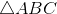has had a rigid motion applied to it to result in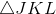?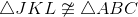Only two angles from each triangle are congruent

Only corresponding angles are congruent

Both corresponding angles and sides are congruent

Both corresponding angles and sides are congruent

Explanation:

Recall that a rigid motion preserves the distance from points within a shape in the plane. Therefore, ifhas had a rigid motion applied to it to result inthat means that all corresponding sides and angles of these two triangle are congruent.

Therefore, the statement "both corresponding angles and sides are congruent" is the correct solution to this particular question.

### Example Question #61 : Congruence

Determine whether the statement is true or false:

Two triangles that are congruent have corresponding points that are separated five spaces. These two triangles describe a rigid motion.

False

True

True

Explanation:

Recall that a rigid motion preserves the distance from points within a shape in the plane. Since the statement clearly states that the two triangles are congruent, that means that the distances between the points within the shape are preserved. Also, since the corresponding points between the two triangles are separated by five spaces this is describing a translation which when combined with the preserved shape, describes a rigid motion. Therefore, the statement is true.

### Example Question #3 : Definition Of Congruency For Triangles: Ccss.Math.Content.Hsg Co.B.7

When a rigid motion is done onto A, C is the resulting image. Are triangles A and C congruent?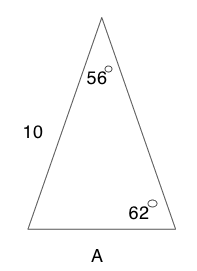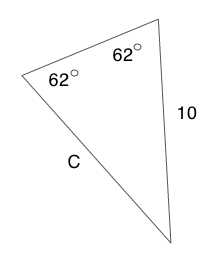Yes

No

Yes

Explanation:

Recall that a rigid motion preserves the distance from points within a shape in the plane. It appears that the rigid motion was a rotation. The third angle can be identified by subtracting the two known angles from 180 degrees.

Triangle A: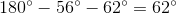Triangle C: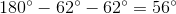Since the side between two angles on triangle C is congruent to the side between those same two angles on triangle A, the two triangles are congruent.

### Example Question #3 : Definition Of Congruency For Triangles: Ccss.Math.Content.Hsg Co.B.7

Determine whether the statement is true or false.

An equilateral triangleis rotated counter clockwise and transformed into an isosceles triangle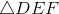is congruent to.

False

True

False

Explanation:

To determine whether this statement is true or false, first recall what it means to be "congruent". Congruent means equal or in more mathematical terms, both corresponding angles and sides of the two triangles are congruent.

The statement says thatis an equilateral triangle which means all sides and angles are the same. After the triangle goes through a transformation, it becomes an isosceles triangle. To be isosceles means that two of the sides and angles of the triangle are the same. Since, not all of the angles are the same init is not congruent to.

Therefore, the statement, "is congruent to." is false.

### Example Question #1 : Definition Of Congruency For Triangles: Ccss.Math.Content.Hsg Co.B.7

Which of the following geometric theorems cannot prove triangle congruency?

Angle, Angle, Angle

Side, Angle, Side

Hypotenuse, Leg of Right Triangle

Side, Side, Side

Angle, Side, Angle

Angle, Angle, Angle

Explanation:

To determine which theorem cannot prove triangle congruency, first recall what it means to be "congruent". Congruent means equal or in more mathematical terms, both corresponding angles and sides of the two triangles are congruent.

There are five theorems used in geometry to prove whether two triangles are congruent.

1. Side, Side, Side

2. Side, Angle, Side

3. Angle, Side, Angle

4. Angle, Angle, Side

5, Hypotenuse and One Leg from Right Triangle

Of the answers below, Angle, Angle, Angle (AAA) is not among one of the theorems to prove triangle congruency. The reason AAA does not prove triangle congruency because two triangles can have the same angles but have different side lengths thus, the triangles would not be congruent.

### Example Question #1 : Definition Of Congruency For Triangles: Ccss.Math.Content.Hsg Co.B.7

Which theorem can be used to prove triangle congruency between triangle A and C?HL

SSS

AAS

SAS

AAA

AAS

Explanation:

For this particular problem there are two geometric theorems that can prove that the triangles are similar.

The geometric theorems that could be used:

1. Angle, Side, Angle (ASA)

2. Angle, Angle, Side (AAS)

For triangle C the AAS is the most evident to use. Since ASA is not an option in the answer selections, AAS is the correct answer.

### Example Question #1 : Definition Of Congruency For Triangles: Ccss.Math.Content.Hsg Co.B.7

Which theorem can be used to prove triangle congruency between triangle A and C?ASA

HL

SSS

AAA

SAS

ASA

Explanation:

For this particular problem there are two geometric theorems that can prove that the triangles are similar.

The geometric theorems that could be used:

1. Angle, Side, Angle (ASA)

2. Angle, Angle, Side (AAS)

For triangle C the AAS is the most evident to use. Since AAS is not an option in the answer selections, ASA is the correct answer.

### Example Question #8 : Definition Of Congruency For Triangles: Ccss.Math.Content.Hsg Co.B.7

Which theorem can be used to prove triangle congruency between triangle A and B?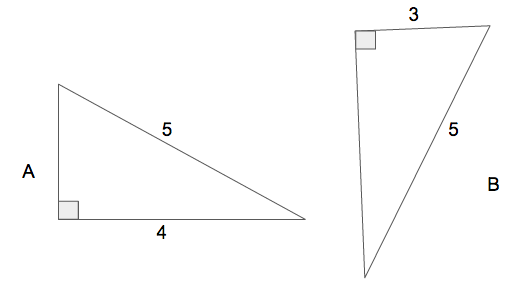SAS

HL

AAS

ASA

AAA

HL

Explanation:

For this particular problem there are two geometric theorems that could potentially be used to prove that the triangles are similar.

The geometric theorems that could be used:

1. Hypotenuse and One Leg of a Right Triangle (HL)

2. Side, Side, Side (SSS)

To use SSS or HL, the Pythagorean theorem will need to be used to calculate the missing side.

For these triangles the HL is the most evident to use and since SSS is not an option in the answer selections, HL is the correct answer.

### Example Question #9 : Definition Of Congruency For Triangles: Ccss.Math.Content.Hsg Co.B.7

Which theorem can be used to prove triangle congruency between triangle A and B?ASA

AAA

SAS

AAS

SSS

SSS

Explanation:

For this particular problem there are two geometric theorems that could potentially be used to prove that the triangles are similar.

The geometric theorems that could be used:

1. Hypotenuse and One Leg of a Right Triangle (HL)

2. Side, Side, Side (SSS)

To use SSS or HL, the Pythagorean theorem will need to be used to calculate the missing side.

For these triangles the HL is the most evident to use but is not an option in the answer selections, therefore, SSS is the correct answer.

### Example Question #10 : Definition Of Congruency For Triangles: Ccss.Math.Content.Hsg Co.B.7

Identify the missing term in the statement.

The __________ geometric theorem deals with proving congruency among right triangles; specifically when the length of one leg and the length of the hypotenuse are known.

Angle, Angle, Side (AAS)

Side, Angle, Side

Side, Side, Side (SSS)

Hypotenuse and One Leg (HL)

Angle, Angle, Angle (AAA)

Hypotenuse and One Leg (HL)

Explanation:

The statement, "The __________ geometric theorem deals with proving congruency among right triangles; specifically when the length of one leg and the length of the hypotenuse are known." is describing the geometric theorem known as the Hypotenuse and One Leg theorem. When abbreviated this is seen as, HL.

Therefore, the missing term is, Hypotenuse and One Leg (HL)

← Previous 1

### All Common Core: High School - Geometry Resources